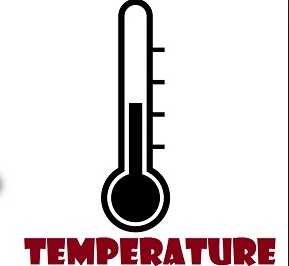# Different methods of temperature measurementIN industries temperature is a major physical quantity . there are different methods of temperature measurement in industries. The different methods of temperature measurement are:
1. Mechanical

2. Electrical.

### 1.Mechanical methods:

#### Mercury in glass thermometers

This consists of a glass tube of very fine bore joined to a reservoir at the bottom and sealed at the top. A measured quantity of mercury is the enclosed. When the thermometer is heated the mercury expands much more than the
glass and is therefore forced to rise up in the tubing A scale is fixed at the side.

####Two metals whose coefficient of linear expansion is different are welded and rolled together to the desire thickness. The actual movement of a bimetal is its flexibility with one end fixed, a straight bimetal strip deflects in proportion to
its temperature, to the square of its length and inversely with its thickens.

#### Compensated Thermometer System:

Compensations are provided in order to nullify the effect of changes in ambient temperature. The compensation in liquid filled expansions thermal system consists of the second tubing and helical element, both liquid filled.
The two elements are so constructed that the measuring helical floats on a movable base the position of which is governed by the compensating helical. The two tubing and helical are matched in volume so that variation in
temperature at the instrument case and along the capillary tubing, produce equal motion from both helical. Such motion nullity each other so that only motion produced by varying the bulb temperature actuates the recorder pen.

####This type depends upon the increase in pressure of a confirm gas (constant volume) due to temperature increase. The relation between temperature and pressure in this kind of system follow Charles law and may be expressed.
The system is filled under high pressure. The increase pressure for each degree of temperature rise is therefore greater than if the filling pressure were low. Nitrogen the gas most after used for such systems, because it chemically
insert and possesses a favorable coefficient thermal expansion.

#### Vapour – Pressure Thermometers:

Vapour pressure thermometers depend upon vapour pressure of liquid, which only partially fills the system. At low temperatures the vapour pressure increase for each unit temperature charge is small, at higher temperature the
vapour pressure change is much greater.

### 2.Electrical method of temperature measurement

#### Thermocouples

It is a simple device consisting of a dissimilar metal wires joined at their ends. When an of each wire is connected to a measuring instrument thermocouples becomes an accurate and sensitive temperature measuring deviceTo know about thermocouple transmitter calibration

### Thermocouple transmitter calibration

#### Resistance – Temperature Detectors (RTD)RTD’s are generally used for precise temperature measurement. It consists of a five wire wrapped around an insulator and enclosed in a metal. The most sheath of a resistance thermometer resembles that of bimetallic thermometer
bulb.

PRINCIPLE: “Resistance increases as temperature increase”
Rt. = Ro (1 + α t )
Rt. = Resistance of Temperature to measured.
Ro. = Resistance of zero temperature.
α    = Co. off of thermal (expansion).
t     = Temperature to be measured.

These metals have a positive temperature co-efficient of expansion. Therefore resistance increases as the temperature increases.
Types of material used: (1) Platinum (2) Nickel
These metals have a positive temperature co-efficient of expansion. Therefore resistance increases as the temperature increases.

Calculation of Resistance or Pt100.
Ro = 100
x for platinum = 0.00385 /c.
To calculate Resistance at 100’c.
R100 = 100 [ 1+ ( 38.5  x 10  4  x 100 ) ]
= 100 + (100  x  0.385)
R100  = 138.5
Resistance at 100’c = 138.5